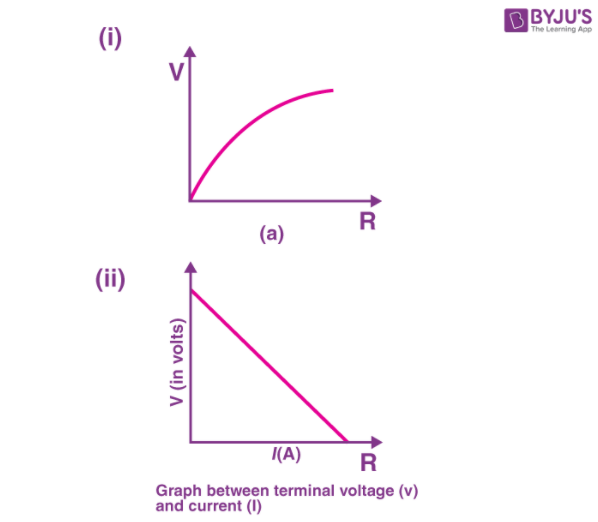# A cell of emf 'E' and internal resistance 'r' is connected across a variable load resistor R. Draw the plots of the terminal voltage V versus (i) R and (ii) the current i. It is found that when R=4Ω, the current is 1A when R is increased to 9Ω, the current reduces to 0.5 A. Find the values of the emf E and internal resistance rWhen R = 4 Ω and I = 1 A

We know that,

Terminal Voltage, V = E – Ir

So we have,

V = IR = 4 = E – Ir

or

E – r = 4 —– (1)

When R’ = 9 Ω and I’ = 0.5 A

V = IR = 0.5 x 9 = E – 0.5r

or

E – 0.5r = 4.5 —– (2)

By solving equation (1) and (2), we get,

r = 1 Ω and E = 5 V

Hence, the value of emf E = 5V and internal resistance r = 1Ω(1)(0)